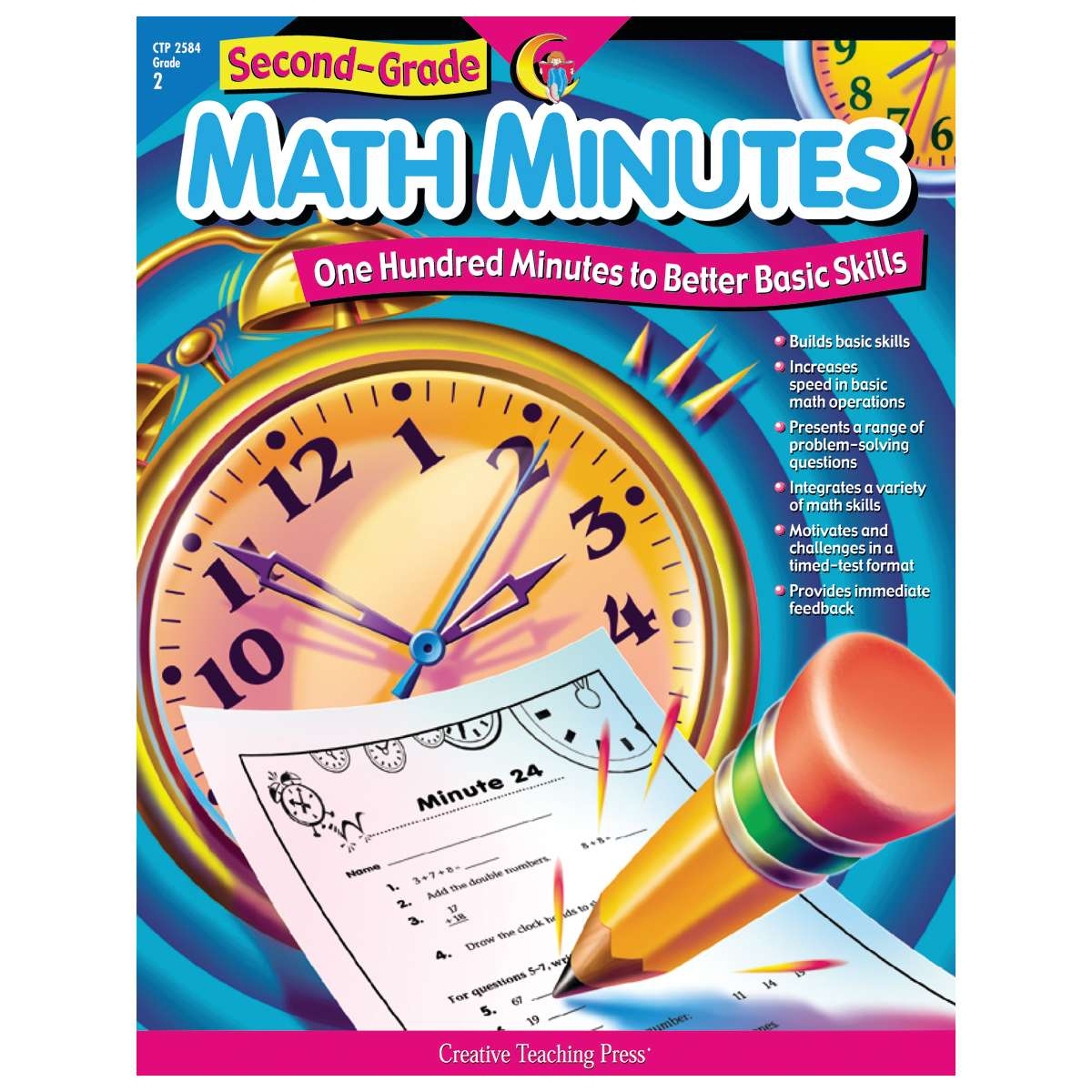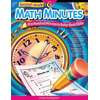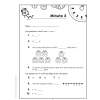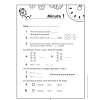•••Math Minutes, Grade 2

Product Number: CTP2584
Each book in this series features 100 “Minutes” to help students build basic skills, increase speed in math operations, and s...
Quantity

Each book in this series features 100 “Minutes” to help students build basic skills, increase speed in math operations, and strengthen problem-solving skills. Each Minute consists of 10 problems of varying degrees of difficulty that incorporate a variety of skills. Each ten-problem reproducible can be used as a learning or testing tool.

Now there's a way for students to practice their math skills in a timed situation, enhance their overall math fluency, and have fun at the same time! Second-Grade Math Minutes provides students with practice in every key area of second-grade math instruction, including:

• skip counting
• doubling numbers
• place value
• three-digit numbers
• fractions
• fact families
• subtraction
• money
• calendar
• graphs
• symmetry
• multiplication
• perimeter
• plane and solid figures
• telling time
• measurement
• and more!

Second-Grade Math Minutes features 100 "Minutes." Each Minute consists of ten classroom-tested problems of varying degrees of difficulty, integrating problem solving and basic math skills. This unique format offers students an ongoing opportunity to improve their own fluency in a manageable, nonthreatening format. The quick, one-minute format combined with instant feedback makes this challenging and motivational assignment students will look forward to each day. Students become active learners as they discover mathematical relationships and apply acquired understanding to complex situations and to the solution of realistic problems in each Minute.

CTP2584